# Plot Background Color in R

Sheeraz Gul May 17, 2022

The background color for the plot can be changed based on the requirements in R. This tutorial demonstrates how to change the plot background color in R.

## Plot Background Color in Base R

`par(bg ="")` is used to change the background color in base R. Let’s try an example.

``````Demo <- rnorm(1000)

# grey background color
par(bg = "grey")

# plot the graph
boxplot(Demo, col = "White")
``````

The code above used `par` to change the background color of the plot in R. The default color is `white`.

We changed it to `grey`. See output plot: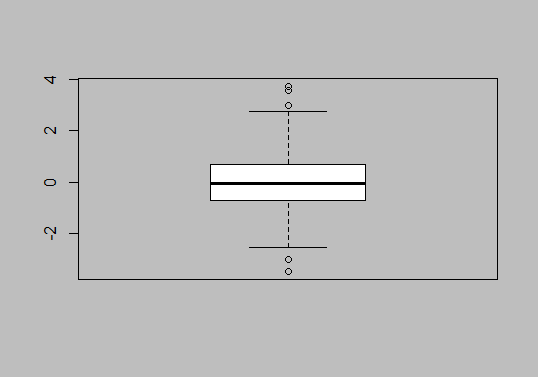As we can see, it changes the background color of the whole plot. To change the background color of the plot region, use this:

``````Demo <- rnorm(1000)

# the basic plot
boxplot(Demo)

# the plot region color
rect(par("usr"), par("usr"),
par("usr"), par("usr"),
col = "grey")

par(new = TRUE)

# Create final plot
boxplot(Demo, col = "white")
``````

This code will select the region of the graph and change the background color. See output: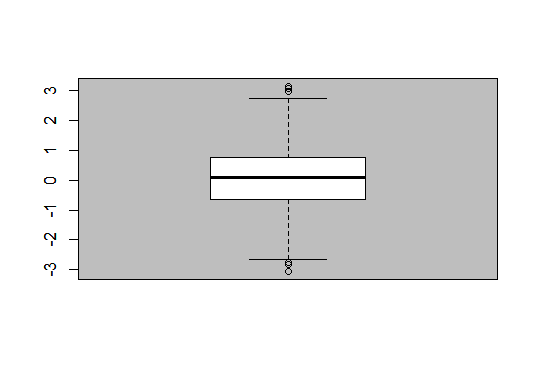Finally, we can also change the background color and plot area color differently. See example:

``````Demo <- rnorm(1000)

# Whole background color
par(bg = "lightblue")

# the basic plot
boxplot(Demo)

# the plot region color
rect(par("usr"), par("usr"),
par("usr"), par("usr"),
col = "grey")

par(new = TRUE)

# Create final plot
boxplot(Demo, col = "white")
``````

The code above will add two colors to the plot; one is the whole background color, and the other is the plot region background color. See output: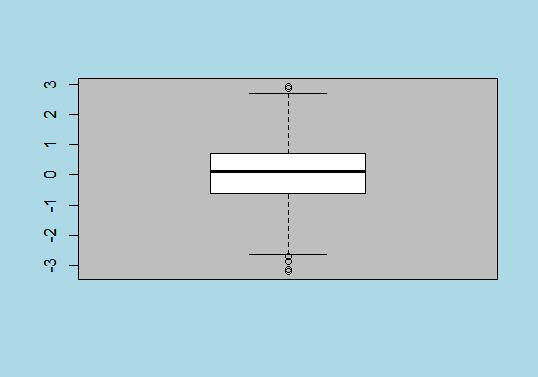## Plot Background Color in `ggplot2` in R

`ggplot2` is the library to plot graphs in R. Let’s first plot a demo graph with `ggplot2` and then try to change the background color.

First of all, load the `ggplot2` library and plot a graph.

``````library("ggplot2")

# Create demo data
demo_data <- data.frame(x = 1:50, y = 1:50)

# plot with ggplot2
ggplot(demo_data, aes(x, y)) + geom_point()
``````

The code above will create a default graph as shown in the output; now, we will try to change the background color.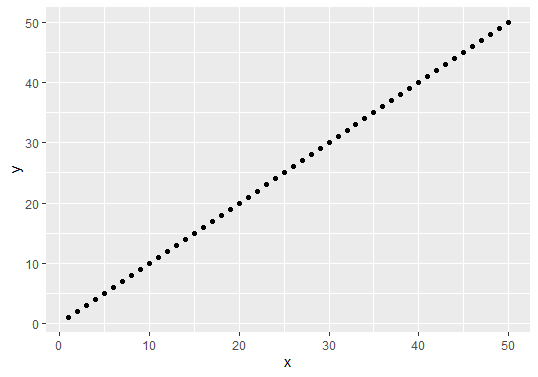Now let’s try to change the background color of the plot region in the above graph. See example:

``````library("ggplot2")

# Create demo data
demo_data <- data.frame(x = 1:50, y = 1:50)

# plot with ggplot2
ggplot(demo_data, aes(x, y)) +
geom_point()+
theme(panel.background = element_rect(fill = "lightblue" ))
``````

The code above will change the background color of the graph region to light blue. See output: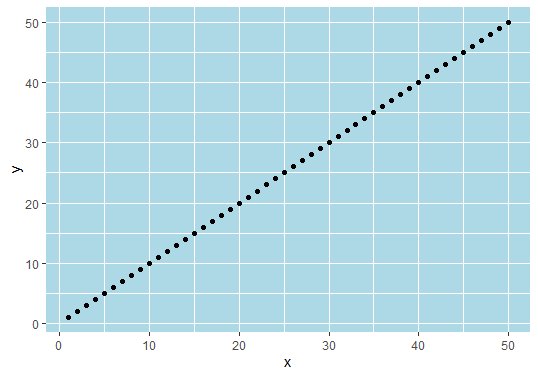Finally, let’s try different background colors and default plot region colors. See example:

``````library("ggplot2")

# Create demo data
demo_data <- data.frame(x = 1:50, y = 1:50)

# plot with ggplot2
ggplot(demo_data, aes(x, y)) +
geom_point()+
theme(plot.background = element_rect(fill = "lightblue" ))
``````

The code above will only change the background color of the graph, not the plot region. See output: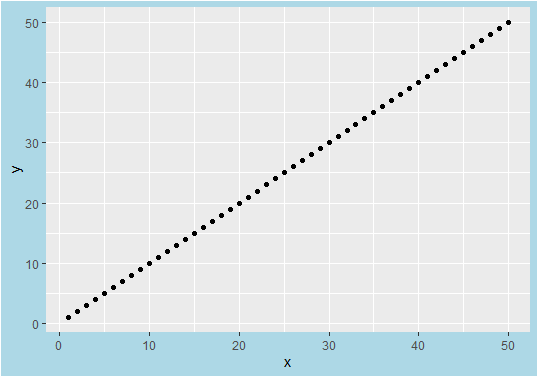Author: Sheeraz Gul

Sheeraz is a Doctorate fellow in Computer Science at Northwestern Polytechnical University, Xian, China. He has 7 years of Software Development experience in AI, Web, Database, and Desktop technologies. He writes tutorials in Java, PHP, Python, GoLang, R, etc., to help beginners learn the field of Computer Science.